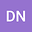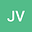Ultra-Compact accurate wave functions for He-like and Li-like iso-electronic sequences and variational calculus. III. Spin-quartet state of the Lithium sequence
•••• +1
Author ProfileJuan Carlos del Valle
Universidad Nacional Autonoma de Mexico Instituto de Ciencias Nucleares
Author ProfileJuan Carlos Lopez Vieyra
UNAM
Author Profile## Abstract

As a continuation of Part I, dedicated to the ground state of He-like and Li-like isoelectronic sequences for nuclear charges $Z \leq 20$, and Part II, dedicated to two excited states of He-like sequence, two ultra-compact wave functions in the form of generalized Guevara-Harris-Turbiner functions are constructed for Li-like sequence. They describe accurately the domain of applicability of the Quantum Mechanics of Coulomb Charges (QMCC) for energies (2-3 significant digits (s.d.)) of the spin-quartet state $1^40^+$ of Li-like ions (in static approximation with point-like, infinitely heavy nuclei). Variational parameters are fitted in $Z$ by 2nd degree polynomials. The most accurate ultra-compact function leads to the absolute accuracy $\sim 10^{-3}$\,a.u. for energy, and $\sim 10^{-4}$ for the normalized electron-nuclear cusp parameter for $Z \leq 20$. Critical charge $Z=Z_B$, where the ultra-compact trial function for the $1^40^+$ state looses its square-integrability, is estimated, $Z_B(1^4\,0^+) \sim 1.26 - 1.30$. As a complement to Part I, square integrability for the compact functions constructed for the {\it ground, spin-doublet state} $1^2\,0^+$ of the Li-like sequence is discussed. The critical charge, for which these functions stop to be normalizable, is estimated as $Z_B( 1^2\,0^+) = 1.62 - 1.65$. It implies that at $Z=2$ - the negative helium ion He$^-$ - both states $1^2\,0^+$ and $1^4\,0^+$ exist as states embedded to continuum.
14 Feb 2022Submitted to International Journal of Quantum Chemistry
19 Feb 2022Submission Checks Completed
19 Feb 2022Assigned to Editor
09 Mar 2022Reviewer(s) Assigned
09 Mar 2022Review(s) Completed, Editorial Evaluation Pending
09 Mar 2022Editorial Decision: Revise Minor